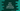# Runes in dart explanation with example# Runes in dart :

Every character, digit, and symbol is defined by a unique numeric value in the computer system. It is called Unicode. It is unique and adopted by almost all modern systems. Using Unicode, data transfer became easy. For example, if you are sending one smiley on a chat app, it will send the Unicode value of that smiley. The other device will read this value and show it as a smiley.

Dart string is a sequence of UTF-16 code units. Dart string has codeUnitAt and codeUnits properties to get these 16 bit code units. Each character in string can be represented by one or multiple code points. Runes is used for Unicode 32-bit values. String class has one property called runes that returns an iterable of Unicode code points of this string. Using the Runes constructor, we can create one runes from a string.

A Unicode is represented in hexadecimal value. It can be expressed as either \uXXXX if it is a four-digit hexadecimal value or the hexadecimal value is placed under {} if it is more than four-digit.

## Example of codeUnits :

``````main(List<string> args) {
var str = "hello \u{1f5fa}";

print(str);
print(str.codeUnits);
}``````

### Output :

``````hello 🗺
[104, 101, 108, 108, 111, 32, 55357, 56826]``````

It is a list of UTF-16 code units.

## codeUnitAt :

``````main(List<string> args) {
var str = "hello \u{1f5fa}";

for (int i = 0; i < str.length; i++) {
var char = str[i];
print("code unit for \${char} is \${str.codeUnitAt(i)}");
}
}``````

It will print the output like below :

``````code unit for h is 104
code unit for e is 101
code unit for l is 108
code unit for l is 108
code unit for o is 111
code unit for   is 32
code unit for ��� is 55357
code unit for ��� is 56826``````

Here, the world map character is represented by two code units.

## Using runes :

``````main(List<string> args) {
var str = "hello \u{1f5fa}";

print(str.runes.toList());
}``````

### Output :

``[104, 101, 108, 108, 111, 32, 128506]``

The hexadecimal value of 128506 is 1f5fa.

## Unicode to string :

We can use the constructor of Runes to find out the string representation from unicode :

``````main(List<string> args) {
var str = "\u0068\u0065\u006C\u006C\u006F \u{1f5fa}";
Runes charCodes = new Runes(str);

print(String.fromCharCodes(charCodes));
}``````

It will print :

``hello 🗺``